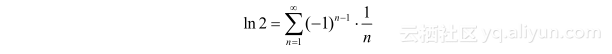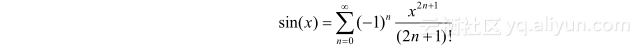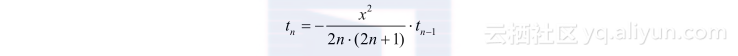《从问题到程序：用Python学编程和计算》——第3章 基本编程技术 3.1 循环程序设计

+关注继续查看

需要循环的情况

1）需要多次执行类似操作而且操作次数较多，适合用循环处理。对这类情况，采用循环通常能缩短程序。用一段公共代码描述公共操作，更容易检查、维护和修改。

2）需要做一些重复性的操作，但在编程时无法确定操作的次数，结束条件由重复工作中数据的情况决定。这类情况就必须通过循环处理。

3）决定重复控制的因素来自函数的参数或程序的输入，也必须用循环描述。

写循环时需要考虑的问题

• 为了完成重复性计算，需要为循环引进哪些变量？用什么变量控制循环？
• 循环开始前，这些变量应该取什么值（初值问题）？
• 在循环体里（在一次迭代计算中），哪些变量应该更新，其值应该如何变化？
• 在什么条件下结束（或者继续）循环？
• 循环结束后，如何得到所需的结果？

浮点数与循环控制

for i in range(0, 10, 2):
print(i)
i -= 1

from math import sin, cos

x = 0.0
value = 0.0
while x != 3.0:
value += sin(x * x + 1) * cos(x) * 0.03
x += 0.03
# 这时value的值应是所需结果

from math import sin, cos

x = 0.0
value = 0.0
while x < 3.0:
value += sin(x * x + 1) * cos(x) * 0.03
x += 0.03

print(value)

from math import sin, cos

value = 0.0
for n in range(100):
x = n * 0.03
value += sin(x * x + 1) * cos(x) * 0.03

print(value)

简单重复

while True:
n = int(input("Next int:"))
if n < 0:
break
print("Factorial of", n, "is", fact(n))

累积

def fact(n):
prod = 1
for k in range(2, n+1):
prod *= k
return proddef ln_2(num):
ln2 = 0.0
sign = 1
for n in range(1, num + 1):
ln2 += sign * 1/n
sign *= -1
return ln2

for i in range(100, 200, 10):
print("First", i, "term of series for ln 2:", ln_2(i))

First 100 term of series for ln 2: 0.688172179310195
First 110 term of series for ln 2: 0.6886223863178897
First 120 term of series for ln 2: 0.688997874401657
First 130 term of series for ln 2: 0.6893158191755918
First 140 term of series for ln 2: 0.6895885067652056
First 150 term of series for ln 2: 0.6898249580908314
First 160 term of series for ln 2: 0.6900319459942255
First 170 term of series for ln 2: 0.6902146544587359
First 180 term of series for ln 2: 0.690377118712483
First 190 term of series for ln 2: 0.6905225267244215

sum = 0
for i in range(1, 1001):
if i % 7 == 2 and i mod 3 != 0:
sum += i

sum = 0
n = 0

while True:
x = int(input("Next integer: "))
if x % 2 == 0:
sum += x
n += 1
if n == 10:
break

递推

d = f(d, ...)

• 选定循环中使用的递推变量；
• 确定这个（这些）递推变量的初值；
• 确定如何从已知信息（包括递推变量的当前值）计算出各个递推变量的下一个值的方法，用程序语句实现相关计算。

def sqrt(x):
guess = 1.0
n = 0
while abs(guess * guess - x) > 1e-8:
guess = (guess + x/guess)/2
n = n + 1
print(str(n) + "th iteration:", guess)
return guess

求sin函数的值def term(x, n):
t = (-1)**n * x ** (2 * n + 1)
for i in range(2, 2 * n + 2):
t /= i
return t

def mysin(x): # 自己定义的计算sin的函数
sn = 0.0
n = 0
while True:
t = term(x, n)
if abs(t) < 1e-6:
return sn
sn += t
n += 1

>>> mysin(0.0)
0.0
>>> mysin(pi/2)
0.999999943741051
>>> mysin(pi)
-7.727858895417119e-07def mysin(x):
sn = x
t = x
n = 0
while True:
n += 1
t *= - x * x / (2*n) / (2*n + 1)
if abs(t) < 1e-6:
return sn
sn += t

>>> mysin(10*pi)
0.00034023652139616377
>>> mysin(20*pi)
-4562371877.727803

from math import fmod, pi

def mysin(x):
x = fmod(x, 2*pi)
sn = x
t = x
n = 0
while True:
n += 1
t *= - x * x / (2*n) / (2*n + 1)
if abs(t) < 1e-6:
return sn
sn += t

>>> mysin(100*pi + pi/2)
0.999999943741051
>>> mysin(-pi/2)
-0.999999943741051
>>> mysin(-pi)
7.727858895155385e-07

• 程序完成后的测试必须比较充分，不仅应该包括最简单的数据实例（如前面的0，pi/2，pi等），还要选择一些不那么常规，但也可能出现的数据实例。
• 在浮点数计算中，误差的积累有可能使结果完全失去价值。对于实际程序里与浮点数有关的部分，必须针对可能情况做充分的测试。
• 选择测试实例，应该找那些容易判断结果正误，但又能反映测试需要的实例。

判断素数

def is_prime(n):
if n < 2:
return False
k = 2
while k * k <= n:
if n % k == 0:
return False
k += 1
return True

for n in range(6, 201, 2):
for i in range(1, n//2 + 1, 2):
if is_prime(i) and is_prime(n - i):
print(n, "=", i, "+", n-i)
break

3.1.3　输入循环

• 在编程时已经明确知道需要输入的数据的项数情况。循环中只需一项项读入数据，完成输入后结束。这种输入循环完全由程序内部控制，是最简单的循环输入情况，相关编程中没有新问题。当然，这种循环输入也存在一些变形，见下。
• 编程时只知道需要从外部输入一批数据，但并不确知输入数据的具体项数。前面说过，这种情况只能通过循环描述。这里的新问题是如何确定循环应该结束。显然程序执行中应该反复循环，直到使用者认为应该结束的时刻。也就是说，这是一种由程序外部控制的输入循环，应称为由输入控制的循环。

简单输入循环

print("Calculating year's rainfall")
rainfall = 0.0
for i in range(12):
x = float(input("Rainfall of a month: "))
rainfall += x
print("The total amount of the year", rainfall)

【例】假定需要输入一系列数值，求出其中前10项非负数据的平均值。

print("Calculate average of 10 input positives.")
sum0 = 0.0
num = 0
while num < 10:
x = float(input("Next number: "))
if x > 0:
sum0 += x
num += 1
print("Average of 10 positives:", sum0 / num)

输入控制的循环

while True:
data = input(…)
if data是表示结束的特殊输入:
break
正常输入的处理

while True:
item = input("...")
if item == "end":
break
data = float(item)
正常输入的处理

print("Calculating average.")
ss = 0.0
n = 0
while True:
snum = input("Next number ('end' to stop): ")
if snum == "end":
break
ss += float(snum)
n += 1
print("The average of these " + str(n) + " numbers is", ss/n)

name = ""
while name != "no more":
name = input("Hi, friend! What is your name? ")
print("Hello, " + name + "!\n")

while True:
name = input("Hi, friend! What is your name? ")
print("Hello, " + name + "!\n")
yesno = input("Next? (Yes/No): ")
if yesno == "No":
break《Python语言程序设计》——2.14　实例研究：计算距离
1093 0《从问题到程序：用Python学编程和计算》——2.10　若干Python机制及其他
906 01652 0《Python语言程序设计》——2.13　软件开发流程
1318 0C/C++程序编译流程（预处理-&gt;编译-&gt;汇编-&gt;链接）

759 0Python编程：Python2.7的MD5编码问题
Python编程：Python2.7的MD5编码问题
18 0【转】C# 计算程序运行时间
1 //计算程序运行时间(.net1.1 于.net2.0的区别)在.net2.0中提供了Stopwatch类，简单例子  2 using System.Diagnostics;  3  4 private Stopwatch stw = new Stopwatch();  5  6 pri...
600 0《多核与GPU编程：工具、方法及实践》----第2章 多核和并行程序设计 2.1 引言

993 0《并行计算的编程模型》一3.6.4　wait和wait_until

844 0776 0
10059

0

+ 订阅

《2021云上架构与运维峰会演讲合集》

《零基础CSS入门教程》

《零基础HTML入门教程》